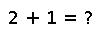# peakflops

``````..  peakflops(n; parallel=false)

``peakflops`` computes the peak flop rate of the computer by using double precision :func:`Base.LinAlg.BLAS.gemm!`. By default, if no arguments are specified, it multiplies a matrix of size ``n x n``, where ``n = 2000``. If the underlying BLAS is using multiple threads, higher flop rates are realized. The number of BLAS threads can be set with ``blas_set_num_threads(n)``.

If the keyword argument ``parallel`` is set to ``true``, ``peakflops`` is run in parallel on all the worker processors. The flop rate of the entire parallel computer is returned. When running in parallel, only 1 BLAS thread is used. The argument ``n`` still refers to the size of the problem that is solved on each processor.``````

## Examples

Checking you are not a robot: